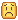# Could I have a __newindex function in a metatable that can also lead to a table being indexed?

What the title says. I want to be able to do something like this:

``````__newindex = function(t, i, v)
if i == "lorem" then
--ipsum
else
return table
end
end
``````

where I can have both a function and an alternate table to be indexed.

I was trying to fix this issue, and I (partially) figured it out.

``````tinst = Instance
Instance = {
new = function(classname)
local tobj = tinst.new(classname)
local obj = setmetatable({
-- custom functions here
}, {
__index = tobj,
__newindex = function(t, i, v)
if i == "Parent" then
if typeof(v) == "table" then
tobj[i] = getmetatable(v).__index
else
tobj[i] = v
end
else
tobj[i] = v
end
end
})

return obj
end
}

local Part = Instance.new("Part")
Part.Parent = workspace
Part.Name = "Okay"

local Rekt = Instance.new("Camera")
Rekt.Parent = game.Lighting
Part.Parent = Rekt
``````
1 Like

Yes that’s alright. For the parent condition, you can condense that to:

``````if i == 'Parent' and typeof(v) == 'table' then
tobj[i] = getmetatable(v).__index
else
tobj[i] = v
end
``````
1 Like

Yes, that is recommended as the “and” operator will do the same as what he is trying to achieve. Also he marked his own post as a solution lol.